### Home > CALC > Chapter 4 > Lesson 4.2.3 > Problem4-79

4-79.
1.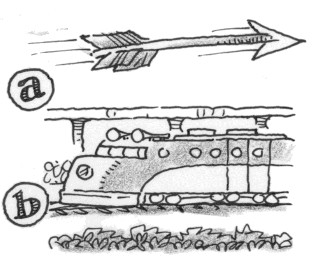Compare how distance and velocity are related with these two scenarios: Homework Help ✎

1.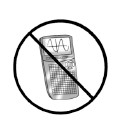As an arrow flies through the air, the distance it has traveled in feet at time t is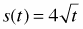. Without your calculator, find the velocity, s'(t), at times t = 1, 4, and 16 seconds. Explain what concepts of calculus you applied in order to solve this problem.

2. As a train travels through a station, its velocity, measured in miles per hour, is v(t) = 9t + 32. If the train is in the station when t = 0, determine the position of the train at t = 1 hour. Explain what concepts of calculus you applied in order to solve this problem.

3. Both (a) and (b) involve distance and velocity. However, each required a different method or approach. Describe the relationship between distance and velocity, as well as the derivative and area under a curve.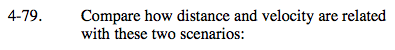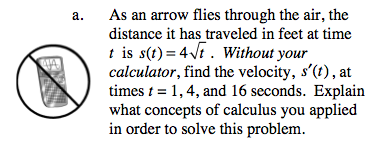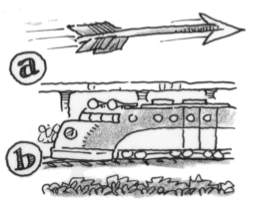Three big concepts to choose from are limits, derivatives and integrals.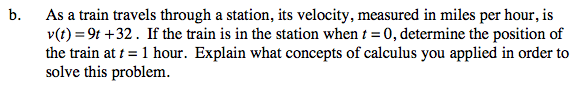Refer to hint in part (a).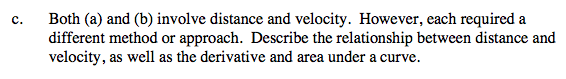Think back to the Freeway Fatality problem 1-1.